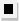Chapter 13.CR, Problem 67E### Algebra and Trigonometry (MindTap ...

4th Edition
James Stewart + 2 others
ISBN: 9781305071742

#### Solutions

Chapter
Section### Algebra and Trigonometry (MindTap ...

4th Edition
James Stewart + 2 others
ISBN: 9781305071742
Textbook Problem

# 6 7 - 6 9Mathematical Induction Use mathematical induction to prove that the formula is true for all natural numbers n . 1 + 4 + 7 + ⋅ ⋅ ⋅ + ( 3 n − 2 ) = n ( 3 n − 1 ) 2

To determine

To prove:

The expression 1+4+7++(3n2)=n(3n1)2 using mathematical induction.

Explanation

Given:

The expression is given as,

1+4+7++(3n2)=n(3n1)2 … (1)

Approach:

Prove basis step for n=1. Assume formula is true for some natural number n1 and show that the formula is true for n+1.

Calculation:

Denote the given expression by P(n).

Step 1:

Substitute n=1 in equation (1).

1=1(311)2

This proves Step 1.

Step 2:

Assume P(n) is true for some n1. This is called the induction hypothesis.

Show that P(n+1) is true.

1+4+7++(3n2)+(3(n+1)2)

can be rewritten using the induction hypothesis as follows

n(3n1)2+(3(n+1)2)=n(3n1)2+3n+1=

### Still sussing out bartleby?

Check out a sample textbook solution.

See a sample solution

#### The Solution to Your Study Problems

Bartleby provides explanations to thousands of textbook problems written by our experts, many with advanced degrees!

Get Started